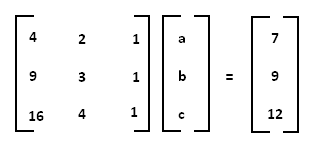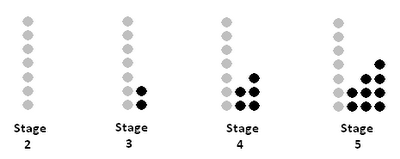## Sunday, September 25, 2011

### Numerous Connections to Quadratic Functions

I think that as secondary math teachers, we ought to be aware of as many ways as possible to model quadratic patterns, so we can seamlessly integrate those techniques into our curricula. It's the first non-straight forward pattern that our kids encounter, and it seems to trouble them throughout their secondary careers. Thankfully, there are ties to quadratic modeling from MANY different topics!

To discuss this concretely, let's first have a look at a quadratic pattern:
(2, 7), (3, 9), (4, 12), (5, 16)

In this discussion, I am going to assume that you've already taught kids how to recognize that this is a quadratic pattern, since that discussion should absolutely precede any quadratic skills, methinks!

• Method #1: Helping kids understand at a middle-school level that we find missing coefficients inside equations by plugging in given x's and y's.

For example, given our points above, y = ax^2 + bx + c becomes:
7 = 4a + 2b + c
9 = 9a + 3b + c
12 = 16a + 4b + c

This we can solve in MIDDLE SCHOOL using systems of equations/elimination method.

• Method #2: When kids get into high school and they start learning about matrices, we can set the same system up as a problem of 3-by-3 matrix, and help them use the calculator to solve it:(It's a good time to make sure everyone understands why we're using inverse matrices, and what identity means in math, etc.)

• Method #3: Of course, now that they're in the realm of graphing calculators, we should also teach them how to use quadratic regression to get the values of the coefficients.

• Method #4: There is another sneakier way of finding the equation using pure quadratics algebra. It's called the Legrange Method, and I learned it this summer at PCMI.

You split the function into smaller, easier quadratic functions by splitting the table and hiding two points at a time:With a bit of simple algebra, you can figure out that
y1 = 3.5(x - 3)(x - 4)
y2 = -9(x - 2)(x - 4)
y3 = 6(x - 2)(x - 3)

Then, when you simplify each and combine them, you'd get your equation for y! This way is not the easiest, but it does reinforce the idea of roots, plugging in numbers to solve for parts of equation, and expansion/simplification of binomials.

• Method #5: Individual elements within a quadratic sequence are created as a summation of arithmetic/linear steps! For example, if you look at the example we have here: (2, 7), (3, 9), (4, 12), (5, 16)

f(2) = 7
f(3) = 7 + 2
f(4) = 7 + 2 + 3
f(5) = 7 + 2 + 3 + 4

Inductively,
f(x) = 7 + { 2 + 3 + 4 + ... +(x-1) }

Or, if we use an arithmetic series formula to represent the sum-of-steps:
f(x) = 7 + [2 + (x-1)][(x-2)/2]

Since my arithmetic series has first term = 2, last term = (x-1), and altogether (x-2)/2 pairs of elements.

• Method #6: There is a name for this trick, but I forget what it is. If we draw out the quadratic sequence as dots, we get:Here I used the gray dots to represent the initial value.

If you double the added dots at each stage (see blue/black dots below), it now becomes instantly easier to find the pattern, because the length and the width of the resulting rectangle is a simple linear progression in terms of x:f(x) = initial value + half of rectangular area
f(x) = 7 + 0.5(length times width of rectangle)
f(x) = 7 + 0.5(x + 1)(x - 2)

• Neat, eh? I think it's pretty amazing how you can approach a simple quadratic pattern from so many different algebraic methods, and I am a true believer that the more of these integrated approaches we take, the better kids will understand each individual method and be able to transfer their knowledge.

By the way, an 8th-grader came up to me and made an insightful observation, the day following our quick class discussion about why we need 3 points in order to find a path of trajectory (quadratic function) using Dan Meyer's basketball pictures. She asked me, "We need 3 points to find a quadratic function, but we need at least 4 points to verify that the pattern IS quadratic, right?" and she drew me a table on the board with some first and second differences. Brilliant! Yes - 3 points is enough to find an equation, but that's like saying two points form a line. That's the minimal amount of info needed to get the equation, but as soon as you have redundant data points, you will know if your regression form is actually correct! How astute of her to make that observation!

1.Hi Mimi,

Even if you have n points (and say n > 3) to which you have fit a quadratic function, you still cannot rule out that the actual regression function is a degree-n polynomial.

Daniel

2.Hi Dan,

Yep - but at some point you have to look at the utility of teaching that to young kids (like in high school). It's much less confusing to let them think that n points probably will fit into a regression function LESS than n-degrees. I think the stretch into understanding that n points CAN be regressed into an n-polynomial is a small one, AFTER they first understand how to find smaller functions like quadratics and cubics and quartics. :) The problem is that most kids never get past the quadratic part, let alone thinking about n-degree polynomial!

Cheers (and hope you are well!),
Mimi

3.Hi Mimi,

Oh, I agree -- I'm just commenting on the prospects of verifying if a function is quadratic using four or more points (i.e. the observation your student made). You can potentially rule out a quadratic (if the fourth point doesn't fit), but you cannot confirm with certainty that it is a quadratic, regardless of how many redundant points you have. I think this kind of asymmetry can be useful to know.

Daniel

4.Hi Mimi,

I had never use the Lagrange method with quadratics too. All of this is very interesting.

Thank you !

5.The Lagrange method also offers an explanation of why any "other" matching polynomial would need to be degree-n, because you could add a secret y4 that was always outputting zero. Then (for your data)

y4 = A(x-2)(x-3)(x-4)

where A can be any number or even another function.

I often ask "What is the smallest-degree polynomial we can fit this data to?"

You also forgot Method #7, finite differences ;)

Good stuff!

6.In fact I've taught this using finite differences...

Given the points (2, 7), (3, 9), (4, 12), (5, 16), you can see the y-values increasing: 7, 9, 12, 16. The first differences of these values are 2, 3, and 4, so we can say that the second difference will be 1. This means that the function is quadratic and has a leading term of (1/2)x^2.

The points for (1/2)x^2:
(2, 2), (3, 4.5), (4, 8), (5, 12.5)

Original points:
(2, 7), (3, 9), (4, 12), (5, 16)

Make the connection to (1/2)x^2

x= 2, y= 7 = 2 + 5
x=3, y= 9 = 4.5 + 4.5
x=4, y= 12 = 8 + 4
x=5, y= 16 = 12.5 + 3.5

Put some parts together and you have:
x= 2, y= 7 = 0.5(2)^2 + 6 - 0.5(2)
x=3, y= 9 = 0.5(3)^2 + 6 - 0.5(3)
x=4, y= 12 = 0.5(4)^2 + 6 - 0.5(4)
x=5, y= 16 = 0.5(5)^2 + 6 - 0.5(5)

So, y = 0.5x^2 + 6 - 0.5x.

Side note: I believe solving linear systems is considered a high school thing in the USA.

Joe